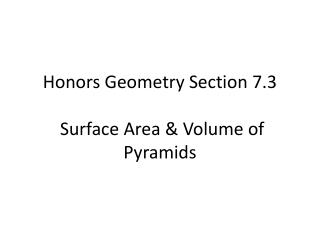# Honors Geometry Section 7.3 Surface Area & Volume of Pyramids - PowerPoint PPT PresentationDownload PresentationHonors Geometry Section 7.3 Surface Area & Volume of Pyramids

Honors Geometry Section 7.3 Surface Area & Volume of Pyramids
Download Presentation## Honors Geometry Section 7.3 Surface Area & Volume of Pyramids

- - - - - - - - - - - - - - - - - - - - - - - - - - - E N D - - - - - - - - - - - - - - - - - - - - - - - - - - -
##### Presentation Transcript

1. A pyramid is a 3-dimensional object consisting of 1 base, which must be a polygon, and three or more lateral faces which are triangles. The lateral faces share a single vertex called the ______ of the pyramid. Base edge and lateral edge are defined in the same way they were for prisms. vertex

2. vertex lateral edge lateral face base edge base

3. The altitude of a pyramid is the segment from the vertex perpendicular to the base.The height of the pyramid is the length of the altitude.

4. The length of an altitude of a lateral face (i.e. the altitude of a triangular face) is called the slant heightof the pyramid. altitude or height Slant height

5. A regular pyramid is a pyramid whose base is a regular polygonand whose lateral faces are congruent isosceles triangles. In a regular pyramid the altitude intersects the base at its ______ and the slant height intersects the base edge at its ________.

6. You should always assume a pyramid is a regular pyramid unless told otherwise.

7. Example 1: The pyramid of Khufu is a regular square pyramid with a base edge of 776 feet and a height of 481 feet. What is the volume of the pyramid?

8. Consider a regular square pyramid whose slant height is l and whose base edge is s.The area of each triangle ofthe net is _______The lateral area is the sum ofthe lateral faces,or ________= ________ ½ s l 4(½ sl) ½ (4s)l

9. Surface Area of a Pyramid = lateral area + area of the base

10. Example 2: The roof of a gazebo is a regular octagonal pyramid with a base edge of 4 feet and a slant height of 6 feet. Find the area of the roof.

11. Example 3: A regular square pyramid has base edges of 8 m and an altitude of 8 m. Find the surface area and volume of the pyramid.

12. Example 3: A regular square pyramid has base edges of 8 m and an altitude of 8 m. Find the surface area and volume of the pyramid.